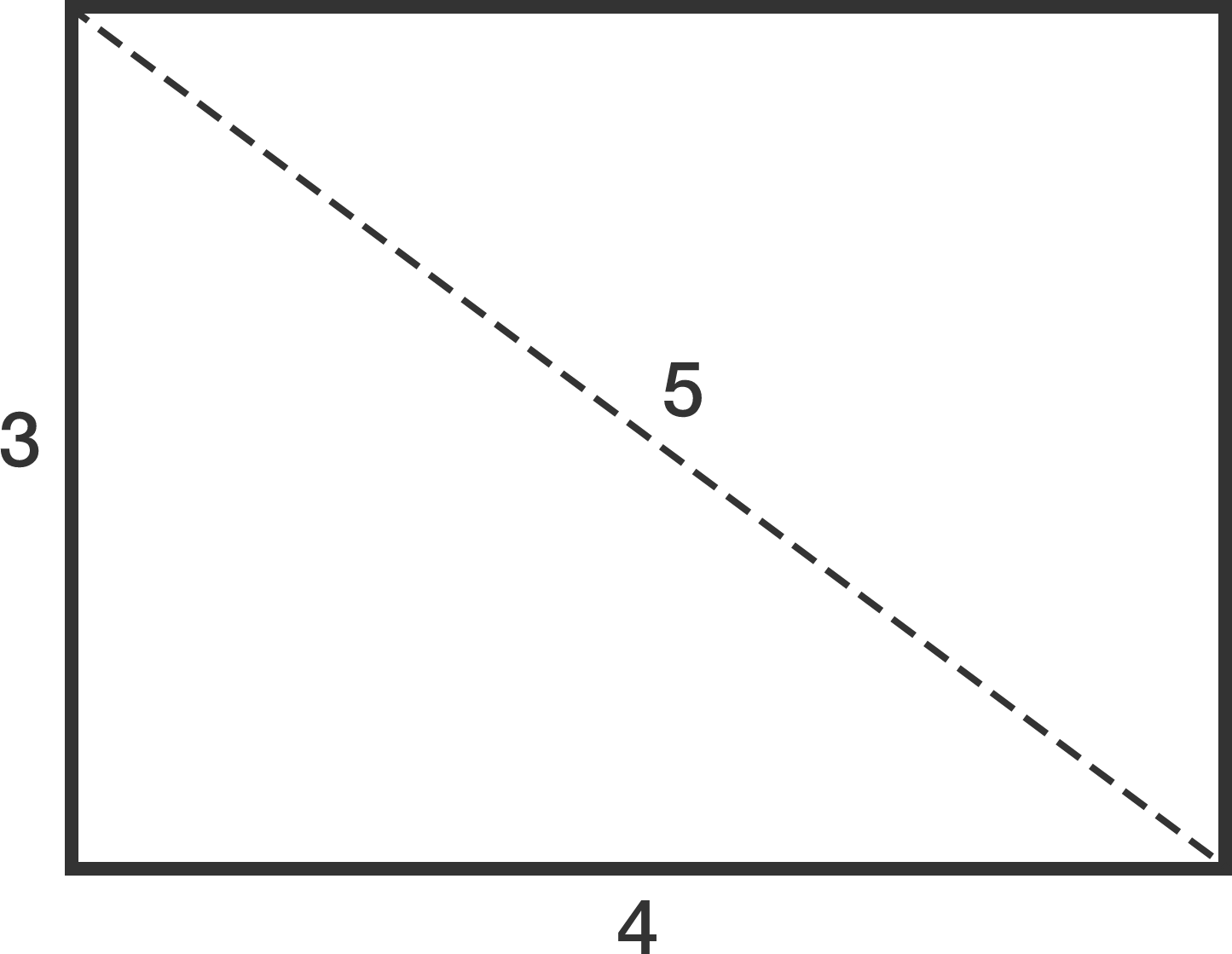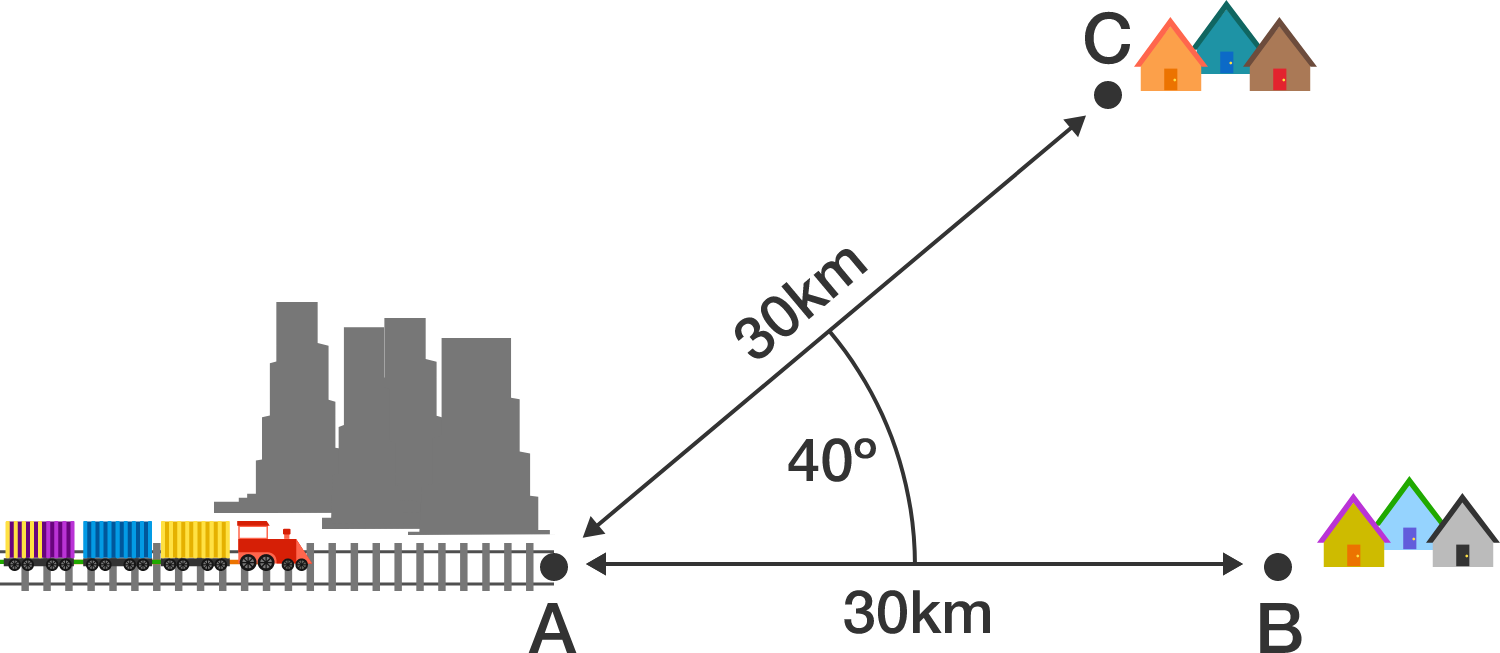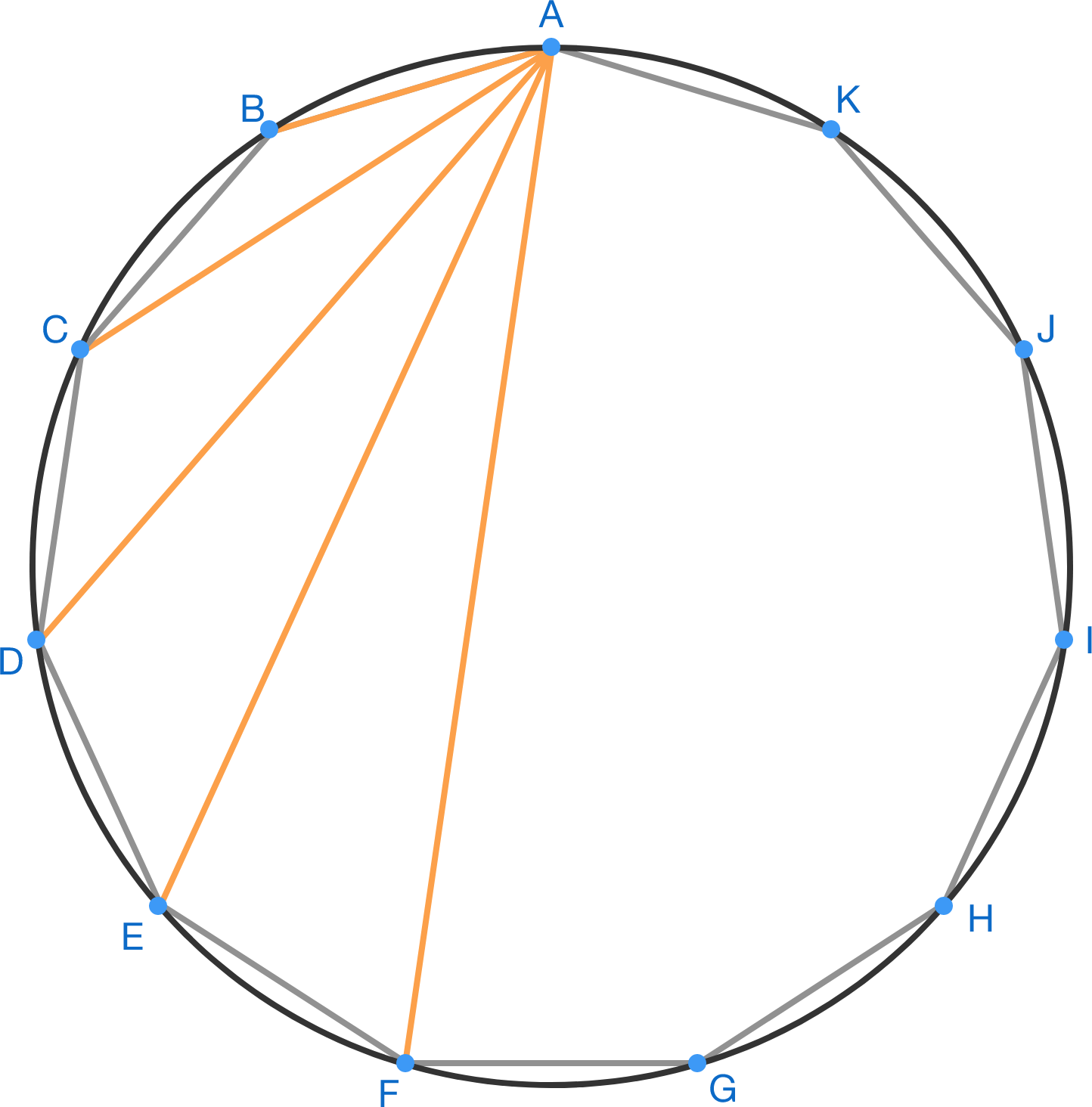# Problems of the Week

Contribute a problemI want to construct a rectangle such that

• it has integer dimensions $a \times b$ that differ by exactly 1 $(\text{i.e. }|a-b|=1);$
• its diagonal also has an integer length.

The diagram to the right shows such a rectangle.

Is there any other way to construct a rectangle satisfying the above properties?

Two villages (points B and C) are to receive a train connection to their neighboring city (point A), as shown in the diagram.In order to save money when building the train route, only the connection with the shortest rail route is selected.

How many kilometers of rails must be laid? Round the result to the nearest integer.

Note: The railroad does not necessarily have to be built on the 30 km segments indicated in the diagram. A railroad switch can be built to allow a railroad to split into different paths.

A regular 11-sided polygon $ABCDEFGHIJK$ is inscribed in a unit circle, as shown below.

If $AB \times AC \times AD \times AE \times AF = \sqrt{k}$

for some positive integer $k,$ find the sum of digits of $k.$The local employment exchange has received 20 applications from 20 different people. They also had 20 different positions (numbered 1 through 20) they could employ people as.

After going through the applications, they made a list of which person is suitable for which position. Below is the list, where each line contains the positions each applicant is suited for.

  1 2 3 4 5 6 7 8 9 10 11 12 13 14 15 16 17 18 19 20 1 3 7 8 9 10 11 12 14 15 17 20 10 11 12 14 15 17 18 20 3 4 6 7 9 13 15 17 20 1 2 6 7 9 10 14 17 5 7 8 11 12 16 17 2 3 4 7 12 17 19 20 1 2 3 4 7 9 11 13 17 1 2 3 8 12 14 17 20 2 3 4 9 10 11 12 13 15 17 18 19 4 11 14 15 16 17 2 7 9 11 13 17 1 2 3 8 14 15 20 1 3 5 7 11 12 17 18 20 1 4 6 8 10 11 12 13 17 18 19 1 5 6 9 15 16 17 18 6 12 13 14 17 18 19 20 2 6 8 10 13 14 15 17 1 3 4 5 6 7 9 10 11 12 13 16 18 20 1 3 5 11 15 18 20 1 7 10 11 12 13 15 

Unfortunately, since each position can be offered to at most one candidate, not all candidates might end up getting a position.

What is the maximum number of people who can each be assigned a position?

Jane, Emily, and Mike are perfect logicians. One day, Jane said, "I'm thinking of four non-negative integers $a, b, x,$ and $y$ that obey the following conditions: \begin{aligned} |a - x| &\geq 1\\ |a - x| &\geq \min(b, y)\\ |a - x| &\leq 1 + \min(b, y)\\ |b - y| &\leq 1. \end{aligned} Then Jane said, "I'm going to tell $a$ and $b$ to Emily and $x$ and $y$ to Mike."

Emily said, "I don't know $x$, and I wouldn't know it even if I knew whether $y$ was the same as $b$."
Mike said, "I don't know $a$, and I wouldn't know it even if I knew whether $b$ was the same as $y$."
Emily said, "I don't know $x$, and I wouldn't know it even if I knew whether $y$ was the same as $b$."
Mike said, "I don't know $a$, and I wouldn't know it even if I knew whether $b$ was the same as $y$."
Emily said, "I don't know $x$, and I wouldn't know it even if I knew whether $y$ was the same as $b$."
Mike said, "I don't know $a$, and I wouldn't know it even if I knew whether $b$ was the same as $y$."

Jane then interrupted, "Stop! You two could go on forever like that!"

Emily said, "I didn't know that."
Mike said, "I didn't know Emily didn't know that. If Emily had said she knew that, I wouldn't know whether Emily knew whether $x$ is greater than $15$. But now, I do."
Emily said, "Before Mike said that, I didn't know whether Mike knew which of $a$ and $x$ is bigger."

What is the maximum value of $a \times b+x \times y?$

×